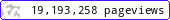•••••MadAsMaths.com :: I.Y.G.B. Practice Papers :: A Level Practice Papers 2019 Spec :: FP3 Practice Papers

I.Y.G.B. FP3 PRACTICE PAPERS

These I.Y.G.B. practice papers mostly follow the Pearson/Edexcel Syllabus introduced for teaching from 2017.

Some modules will be first examined in 2018 and some in 2019.

• Papers A to R have standard difficulty with later papers usually more difficult.
• Papers S and T are extremely hard.
• Papers U to Z are hard.

All papers have model solutions.

 fp3_a.pdffp3_a_solutions.pdffp3_b.pdffp3_b_solutions.pdffp3_c.pdffp3_c_solutions.pdffp3_d.pdffp3_d_solutions.pdffp3_e.pdffp3_e_solutions.pdffp3_f.pdffp3_f_solutions.pdffp3_g.pdffp3_g_solutions.pdffp3_h.pdffp3_h_solutions.pdffp3_i.pdffp3_i_solutions.pdffp3_j.pdffp3_j_solutions.pdffp3_k.pdffp3_k_solutions.pdffp3_l.pdffp3_l_solutions.pdffp3_m.pdffp3_m_solutions.pdffp3_n.pdffp3_n_solutions.pdffp3_o.pdffp3_o_solutions.pdffp3_p.pdffp3_p_solutions.pdffp3_q.pdffp3_q_solutions.pdffp3_r.pdffp3_r_solutions.pdffp3_s.pdffp3_s_solutions.pdffp3_t.pdffp3_t_solutions.pdffp3_u.pdffp3_u_solutions.pdffp3_v.pdffp3_v_solutions.pdffp3_w.pdffp3_w_solutions.pdffp3_x.pdffp3_x_solutions.pdffp3_y.pdffp3_y_solutions.pdffp3_z.pdffp3_z_solutions.pdf